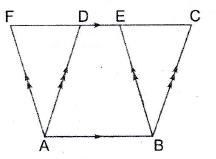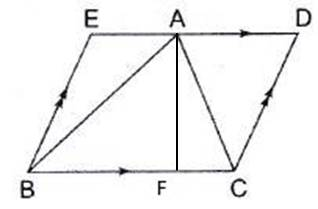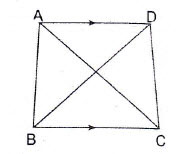Area

The amount of surface enclosed by the sides of a closed bounded figure is called its area.

Definition of Base and Altitude of a Parallelogram

Any side of a parallelogram can be called its base.

The length of the line segment which is perpendicular to the Base from the opposite side is called the altitude or height of the parallelogram corresponding to the given base.

In the adjoining figure we see that,

1.$DL$ is the altitude corresponding to the base$AB$ of parallelogram$ABCD$; and

2.$DM$ is the altitude corresponding to the base$BC$ of the parallelogram$ABCD$.

Area of a Parallelogram: If you see the figure below, then you could cut the left side of figure (blue triangle) and move it to the right side. It will make it a rectangle.

Hence you can calculate the area of the Parallelogram as. This is a pictorial way of coming up with a formula for area of Parallelogram.

There is a very good animation of the above diagram:

Theorem 1: Parallelograms on the same base and between the same parallels are equal in area.

In the adjoining figure,$ABCD \ and\ ABEF$ are two parallelograms. They have the same base$AB$ and are between the same parallels$AB \ and\ FC$. This means that the altitude for both is the same.$\therefore (\parallel gm \ ABCD)=Area(\parallel gm \ ABEF)$

Corollary:  Since a rectangle is also a parallelogram. Therefore we can use the above theorem to say that:A parallelogram and a rectangle on the same base and between the same parallels are equal in area. (Same base x height)

With this we can derive the formula for the area of the triangle.

Consider a parallelogram$ABCD$ and a rectangle$ABEF$ on the same base$AB$ and between the same parallel lines$AB \ and\ FC$. Then,$Area(\parallel gm\ ABCD)=Area(\parallel gm\ ABEF)=AB\times AF (base \times height)$

Hence,$Area \ of \ Parallelogram=base\times height$

Theorem 2: If a triangle and a parallelogram are on the same base and between the same parallels, then$Area \ of \ the \ triangle=\frac{1}{2}(Area \ of \ the \ parallelogram)$

In each of the adjoining figures,$\Delta ABC \ and\ \parallel \ gm\ BCDE$ are on the same base$BC$ and between the same parallels$BC \ and\ AD$ .$\therefore (\Delta ABC)=\frac{1}{2}(Area \ of \ the \ \parallel \ gm \ BCDE)$We can even drive the area of a triangle using this theorem.

Consider a$\Delta ABC$  and on the same base BC and between the same parallels$BC \ and \ AD$.

Then, using the above result, we have:$\therefore Area(\Delta ABC)=\frac{1}{2}\times Area (\parallel \ gm \ ABCD)$$=\frac{1}{2} \times BC \times AL$ [Using the formula of the area of ||gm]But,$AL$ is the height corresponding to base$BC$ of$\Delta ABC$.

Hence,$Area\ of\ Triangle= \frac{1}{2}\times base \times height$.

Theorem 3: Two triangles on the same base and between the same parallels are equal in area.In the given figure, are on the same base$BC$ and between the same parallels$BC \ and\ AD$.$\therefore \ Area(\Delta ABC)=Area(\Delta DBC)$

Theorem 4: Two Triangles having equal base and lying between the same parallels are equal in area.

In the adjoining figure$\Delta ABC \ and\ \Delta DEF$ have equal base$BC=EF=3\ cm$ (or any measurement) and they are between the same parallels$BF \ and \ AD$.

This is because the base is the same and the height (distance between the parallel lines) is the same.$\therefore Area (\Delta ABC)= Area (\Delta DEF)$